[BACK]Computer Modeling in Engineering & SciencesDOI: 10.32604/cmes.2022.019754

ARTICLE

Two-Machine Hybrid Flow-Shop Problems in Shared Manufacturing

Ningbo University of Finance & Economics, Ningbo, 315175, China
*Corresponding Author: Qi Wei. Email: weiqi@nbufe.edu.cn
Received: 13 October 2021; Accepted: 01 November 2021

Abstract: In the “shared manufacturing” environment, based on fairness, shared manufacturing platforms often require manufacturing service enterprises to arrange production according to the principle of “order first, finish first” which leads to a series of scheduling problems with fixed processing sequences. In this paper, two two-machine hybrid flow-shop problems with fixed processing sequences are studied. Each job has two tasks. The first task is flexible, which can be processed on either of the two machines, and the second task must be processed on the second machine after the first task is completed. We consider two objective functions: to minimize the makespan and to minimize the total weighted completion time. First, we show the problem for any one of the two objectives is ordinary NP-hard by polynomial-time Turing Reduction. Then, using the Continuous Processing Module (CPM), we design a dynamic programming algorithm for each case and calculate the time complexity of each algorithm. Finally, numerical experiments are used to analyze the effect of dynamic programming algorithms in practical operations. Comparative experiments show that these dynamic programming algorithms have comprehensive advantages over the branch and bound algorithm (a classical exact algorithm) and the discrete harmony search algorithm (a high-performance heuristic algorithm).

Keywords: Hybrid flow-shop; dynamic programming algorithm; computational complexity; numerical experiments; shared manufacturing

1  Introduction

1.1 Problem Statement

In this paper, we study two two-machine hybrid flow-shop scheduling problems with fixed processing sequences. In this problem, a set of n jobs J={J1,J2,,Jn} is processed in a two-machine two-stage flow-shop with machine M1 at Stage 1 and machine M2 at Stage 2. Each job Ji has two tasks Ai and Bi, where task Ai is flexible which can be processed on either of the two machines (M1 and M2) for ai time units, and task Bi is inflexible which must be processed on M2 for bi time units. Task Bi cannot be processed until task Ai is completed, and pre-emption is not allowed. The processing order of the jobs is given in advance. Without losing generality, we assume that the jobs are processed according to the subscript order, i.e., for any two tasks processed on the same machine, the task with a small subscript must be completed before the task with a large subscript. The objectives are to minimize the makespan (the maximum completion time) and minimize the total weighted completion time.

Hybrid flow-shop problems are widely used in the traditional manufacturing industry , computer graphics processing , medical operation scheduling , and other fields. For the research on the hybrid flow-shop problem, please refer to the latest review . A typical application scenario of the two-stage hybrid flow-shop scheduling is as follows. In integrated circuit manufacturing or mechanical manufacturing, product processing usually includes two stages: preliminary processing and finishing processing. The factories are equipped with two sets of equipment, one set of low-precision equipment for preliminary processing and the other set of high-precision equipment for finishing processing. However, when necessary, the high-precision equipment can also be degraded for preliminary processing. Reasonably arrange the processing sequence and processing machines can make all products complete as soon as possible.

The scheduling problems with fixed processing sequences refer to the scheduling problem in which the processing order of the jobs is determined in advance. This kind of problem is originally mainly applied to the stock control systems . In recent years, with the integration of the sharing economy into the traditional manufacturing industry, there has been a shared manufacturing mode that shares manufacturing resources and demands on the network platform and matches supply and demand through advanced algorithms . “Alibaba Taobao Factory” and “Aerospace Cooperative Manufacturing Network” in China, “Wanju-gun Local Food Processing Center” in Korea, and many other share manufacturing practices have been vigorously developed. Based on fairness, shared manufacturing platforms often require manufacturing service enterprises to arrange production according to the principle of “order first, finish first” which forms a constraint of fixed processing sequences. Suppose the two-stage hybrid flow-shop scheduling problem with two machines described above is considered in the shared manufacturing environment. It is equivalent to adding the constraint of fixed processing sequences in the above typical application scenario. Obviously, the mathematical model of this problem is the scheduling model described in the first paragraph of this section.

1.2 Related Problems and Results

Wei et al.  first considered this two-stage hybrid flow-shop scheduling problem without fixed processing sequences constraint (denoted by SHFS) in 2005. They proved that this problem is ordinary NP-hard and presented an approximation algorithm with polynomial-time computational complexity whose worst-case ratio is 2. For the same problem, Jiang et al.  designed an improved approximation algorithm whose worst-case ratio is 1.5. Recently, for the case where every job has a deadline and must be processed in a given order, Wei et al.  proposed efficient algorithms.

The research results of other hybrid flow-shop scheduling problems with two stages close to the problems studied in this paper are as follows. A special hybrid flow-shop scheduling problem with two machines was considered by Vairaktarakis et al. . In their problem, the two tasks of each job can be processed on any one of the two machines, i.e., there are four processing methods in total. An excellent approximate algorithm was presented whose worst-case ratio is only 1.618. Zhang et al.  studied another similar problem where the later task of each job can be jointly processed by multiple machines, but the former task cannot. An approximate algorithm was presented in their paper whose worst-case ratio is only 2ε. For the special case of the above problem raised by Zhang et al. , an improved approximation algorithm was presented by Peng et al.  respectively studied two hybrid flow-shop problems where all batching machines are at two stages. The objective function of the former problem considered by Wang et al.  is to minimize the total weighted completion time. Mixed-integer linear programming (MILP) was constructed, and an efficient heuristic algorithm was presented. The objective of the latter problem studied by Tan et al.  is to minimize the total weighted delay time. They proposed an iterative decomposition method to solve it. Some scholars have also studied the two-stage hybrid flow-shop where no buffer capacity exists between the two stages. For the latest research results on such problems, please refer to the research of Dong et al. . For other latest studies on two-stage hybrid flow-shop under different parameter conditions and objective functions, please refer to Feng et al. , etc. However, these problems do not consider the constraint of fixed processing sequences.

The earliest scheduling problem with fixed processing sequences was presented by Shafransky et al. . They considered the model with fixed processing sequences in an open-shop problem, showed this problem is NP-hard in the strong sense, and proposed a very accurate polynomial-time approximate algorithm whose worst-case ratio is only 1.25. Lin et al.  studied a two-stage differentiation flow-shop to minimize the total completion time and proposed a dynamic programming algorithm. Hwang et al. [23,24] firstly studied the general two-machine flow-shop problem with a given sequence and presented an optimal algorithm for each of the two special cases. Then, they studied the general flow-shop scheduling with batching machines and proposed optimal algorithms for several models. With the gradual emergence of the application value of the scheduling problem with fixed processing sequences, the related research has increased a lot in recent years. Lin et al.  considered a relocation scheduling problem with fixed processing sequences corresponding to resource-constrained scheduling on two parallel dedicated machines. They proposed polynomial-time optimal algorithms or approximate algorithms for several models with different objective functions. Halman et al.  further gave a fully polynomial-time approximation scheme for the above problem. Cheref et al.  studied a scheduling problem considering production and delivery with a given sequence. They showed that this problem is NP-hard and proposed a dynamic programming algorithm with good effect. A server scheduling problem on parallel dedicated machines with fixed processing sequences was considered by Cheng et al. [28,29]. For the two-machine case, a polynomial-time approximation algorithm was presented; for the case where each loading time is unit, two heuristic algorithms were proposed; and for the general case, a pseudo-polynomial algorithm was presented. However, the two-stage hybrid flow-shop scheduling with fixed processing sequences and the goal of minimizing makespan or total weighted completion time has not been considered yet.

1.3 Our Results

In the “shared manufacturing” environment, we introduce the constraint of fixed processing sequences into the two-machine two-stage hybrid flow-shop scheduling problem SHFS. We consider two objectives of this problem: to minimize the makespan and to minimize the total weighted completion time. For each problem, we analyze the computational complexity and present a dynamic programming algorithm. According to the three-field representation, these two problems can be expressed in the following form:

(1) FS2|FJS,Hybrid|Cmax,

(2) FS2|FJS,Hybrid|wiCi,

which are denoted by SHFSFC and SHFSFW.

Firstly, we give the structural characteristics of one optimal solution and prove that these two problems are both ordinary NP-hard. Then we propose the dynamic programming algorithms and calculate their time complexity. Finally, we show the advantages of these algorithms over the existing exact algorithms and heuristic algorithms in practical efficiency and effect by numerical experiments.

The rest of this paper is arranged as follows: In Section 2, we first give the basic symbolic assumptions and the characteristics of one optimal solution, then analyze the computational complexity of the problems; In Sections 3 and 4, the dynamic programming algorithms for SHFSFC and SHFSFW are proposed, respectively; In Section 5, we compare the dynamic programming algorithms presented in this paper with the existing algorithms through numerical experiments to obtain the actual efficiency and effect of these algorithms. Finally, we conclude the paper and give the future research ideas in Section 6.

2  Symbolic Assumptions, Structure of One Optimal Schedule, and Complexity of the Problems

The basic symbols to be used in the following are given, properties of one optimal schedule of SHFSFC (SHFSFW) are analyzed, and the computational complexity of each problem is proved in this section.

2.1 Symbolic Hypothesis

The symbols and their meanings to be used below are as follows:

•   ai: The processing time of task Ai (the first task of job Ji) on machine M1 or M2;

•   bi: The processing time of task Bi (the second task of job Ji) on machine M2;

•   Ci: The completion time of job Ji;

•   wi: The weight of job Ji;

•   V1: The job set {Ji|Ai is processed on machine M1};

•   V2: The job set {Ji|Ai is processed on machine M2}.

2.2 Structure of Optimal Scheduling

The two problems studied in this paper do not consider the buffer capacity between the two stages. That is, the buffer capacity is deemed to be infinite. So the tasks processed on machine M1 can be processed as early as possible, i.e., there is no idle on M1 until completion. If job JiV1, task Bi cannot be processed until task Ai is completed. So, there may be an idle time on machine M2 before task Bi starts to be processed. But if job JiV2, task Ai and Bi can be processed immediately after task Bi1 on M2 without any idle time.

From the above analysis, it is easy to find that the following proposition holds whether the objective is to minimize makespan or total weighted completion time.

Proposition 2.1 There exists one optimal schedule of SHFSFC (SHFSFW) that satisfies the following properties at the same time:

(1)   Task A1 is processed on M2, and task An is processed on M1;

(2)   M1 has no idle time until processing is complete;

(3)   There is no idle time on M2 between any task of the job in V2 and its previous task;

(4)   There is no idle time on M2 between the second task Bi of job JiV1 and its previous task, or the starting processing time of Bi is exactly equal to the completion time of Ai.

Proof:

(1)   Suppose ϕ1 is an optimal schedule of SHFSFC (SHFSFW) where A1 is processed on M1 and An is processed on M2. We construct a new schedule φ1 based on ϕ1 as follows: Change the processing mode of J1 and Jn, i.e., A1 and B1 are all processed on M2 and An is processed on M1; Keep other task processing arrangements unchanged. Since B1 cannot be processed on M2 until A1 is completed on M1 in ϕ1, there is an idle time of a1 time units before task B1 starts to be processed on M2 in ϕ1. Therefore A1 can be processed on the above idle time on M2 in φ1 without affecting the completion time of B1. And since Jn is the last job, no task is processed on M1 after the starting processing time of An (denoted by S(An)) in ϕ1. So An can be processed on M1 from S(An) to S(An)+an in φ1 without affecting the completion time of Bn on M2. Obviously, φ1 is feasible, and the makespan and the total weighted completion time in φ1 are all equal to them in ϕ1. So we have that φ1 is also an optimal schedule which implies property (1) holds.

(2)   Suppose ϕ2 is an optimal schedule of SHFSFC (SHFSFW) satisfying property (1) where there exists an idle time between two successive processed tasks Ai and Aj on machine M1. Next, we construct a new schedule φ2 based on ϕ2 as follows: Advance the start processing time of task Aj to the completion time of task Ai so that there is no idle time between them; Keep other task processing arrangements unchanged. Since the buffer capacity between two stages is not considered, the task Aj is processed in advance, and task Bj remains intact, the starting processing time of Bj is still larger than the completion time of Aj, i.e., φ2 is still feasible. Considering that the processing schedules of all second tasks have not changed in φ2, the completion time of each job is the same in ϕ2 and φ2. For ϕ2 is an optimal schedule of SHFSFC (SHFSFW), φ2 is also an optimal schedule. On M1, by a similar method, all idle times before M1 finishing processing can be eliminated, and the schedule is still optimal, which implies property (1) and (2) hold simultaneously.

(3)   Suppose ϕ3 is an optimal schedule of SHFSFC (SHFSFW) satisfying property (1) and (2) where there is an idle time on M2 between the task Ai (or Bi) of job JiV2 and its previous task. Next, we construct a new schedule φ3 based on ϕ3 as follows: Advance the start processing time of task Ai (or Bi) to the completion time of its previous task on M2, so that there is no idle time between them; Keep other task processing arrangements unchanged. Since the processing arrangements of the jobs in V1 have not changed, φ3 is feasible. And for the completion time of the job in φ3 is equal to or less than that in ϕ3, the makespan and the total weighted completion time in φ3 are all less than or equal to them in ϕ3. According that ϕ3 is an optimal schedule, φ3 is also an optimal schedule. Obviously, by a similar method, all idle times on M2 between any task of the job in V2 and its previous task can be eliminated, and the schedule is still optimal, which implies property (1), (2), and (3) hold at the same time.

(4)   Suppose ϕ4 is an optimal schedule of SHFSFC (SHFSFW) satisfying property (1), (2), and (3) where there is an idle time on M2 between the second task Bi of the job JiV1 and its previous task, and the starting processing time of Bi is larger than the completion time of Ai. Next, we also construct a new schedule φ4 based on ϕ4 as follows: Advance the start processing time of task Bi to the larger value of the completion time of its previous task on M2 and the one of Ai, so that there is no idle time between Bi and its previous task or the starting processing time of Bi is exactly equal to the completion time of Ai; Keep other task processing arrangements unchanged. Obviously, through a simple analysis similar to the proof of (2) or (3), we can have that φ4 is also an optimal schedule and then get property (1), (2), (3), and (4) hold at the same time.

To sum up, we can get Proposition 2.1 holds.

According to Proposition 2.1, it is easy to obtain there exists an optimal schedule of SHFSFC (or SHFSFW), which is shown in Fig. 1 below. That is, there exists an optimal schedule of SHFSFC (or SHFSFW) in which A1 and An are processed on M1 and M2, respectively, and the continuous processing tasks are divided by some idle times before the second tasks of some jobs in V1 on M2. These continuous processing tasks are called Continuous Processing Modules (denoted by CPM) in the following study, which will help design dynamic programming algorithms in Sections 3 and 4.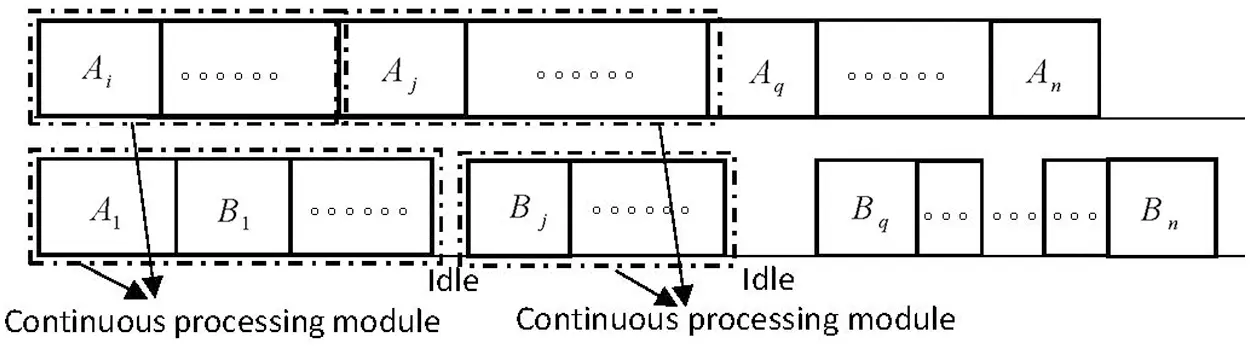Figure 1: The structure of an optimal schedule

2.3 Computational Complexity of the Problems

Next, we prove that SHFSFC and SHFSFW are both NP-hard by Turing Reduction.

Theorem 2.2 Problem SHFSFC is NP-hard, even if the processing time of the second task of every job is 0, i.e., bi=0 for all i.

Proof: First, propose an Instance I of a well-known NP-hard problem Partition Problem: There is a set of n integers E={e1,e2,,en} and an integer C=12i=1nei. Can set E be divided into two disjoint subsets E1 and E2, such that E1E2=,E1E2=E, and eiE1ei=eiE2ei=C?

Then, based on Instance I, we construct an Instance II of SHFSFC: There is a job set of n+2 jobs J={J0,J1,J2,,Jn,Jn+1}, where

(1)   J0: a0=Cε and b0=ε;

(2)   Ji: ai=ei and bi=ε (i=1,2,n);

(3)   Jn+1: an+1=C+nε and bn+1=0 (ε is a number much smaller than C).

Is there a feasible schedule of Instance II that makes makespan Cmax=2C+nε?

Now, let's prove that the solutions of Instances I and II can be derived from each other. Suppose (E1,E2) is a solution of Instance I, i.e., E1 and E2 satisfy E1E2=,E1E2=E, and eiE1ei=eiE2ei=C. Next, we construct the solution of Instance II as follows: Let V1={Ji|eiE1}{Jn+1} and V2={J0}{Ji|eiE2}. It is easy to have Cmax=C+JiV2/{J0}ai+nε=C+eiE2ei+nε=2C+nε, which implies (V1,V2) is a solution of Instance II.

Suppose (V1,V2) is a solution of Instance II. Let's first prove that J0 must belong to set V2, i.e., the task A0 must be processed on M2. If A0 is processed on M1, M2 has an idle time from time 0 to time a0 (=Cε). So, after time Cε, the total processing time of the rest tasks to be processed by two machines is i=0n+1(ai+bi)(Cε)=3C+(2n+1)ε. We can easily get the makespan is at least (Cε)+1/2(3C+(2n+1)ε)=5/2C+nε1/2ε>2C+nε, which is a contradiction with Cmax=2C+nε. Therefore, we have J0V2.

Next, we prove that Jn+1 must belong to set V1, i.e., task An+1 must be processed on M1. If An+1 is processed on M2, no task can be processed on machine M1 after the starting processing time of task An+1. So, before the starting processing time of task An+1, the total processing time of the tasks to be processed by two machines is (4C+2nε)(C+nε)=3C+nε. We can easily get the makespan is at least 1/2(3C+nε)+an+1+bn+1 = 1/2(3C+nε)+C+nε5/2C+3/2nε>2C+nε which is a contradiction with Cmax=2C+nε. Therefore, we have Jn+1V1.

Considering that Cmax=2C+nε and the total processing time of all jobs is 4C+2nε, it is easy to have that each machine's load is 2C+nε. And since Jn+1V1, the total processing time of the tasks processed on M1 except An+1 is 2C+nε(C+nε)=C, i.e., we have JiV1/{Jn+1}ai=JiV1/{Jn+1}ei=C. Similarly, we can have that the total processing time of the tasks processed on M2 except A0 and the second tasks of all jobs is 2C+nεCnε=C, i.e., we have JiV2/{J0}ai=JiV2/{J0}ei=C. Now we let E1={ei|JiV1/{Jn+1}} and E2={ei|JiV2/{J0}}. Obviously, we have eiE1ei=C and eiE2ei=C, which implies (E1,E2) is a solution of Instance I.

It is known that Partition Problem is NP-hard, so it is easy to have that SHFSFC is also NP-hard.

Obviously, the above proof process still holds when ε=0, so it can be obtained that Theorem 2.2 holds even if the processing time of the second task for every job is 0.

Theorem 2.3 SHFSFW is NP-hard.

Proof: Considering that the jobs are processed in subscript order, we have Cmax=Cn. So when w1=w2==wn1=0,wn=1, problem SHFSFC is a special case of problem SHFSFW. We have proved that SHFSFC is NP-hard, so SHFSFW is also NP-hard.

3  A Dynamic Programming Algorithm of Problem SHFSFC

According to Proposition 2.1, there is an optimal schedule of Problem SHFSFC, which is composed of several continuous processing modules and the idle times between continuous processing modules. Therefore, the following dynamic programming algorithm includes two stages: Construct the optimal continuous processing modules and connect the optimal continuous processing modules through idle times to form an optimal schedule. Firstly, the strict definition of continuous processing module for SHFSFC is given:

Definition 3.1 A sub-schedule consisting of a job subset {Ji,Ji+1,,Jj} is called a continuous processing module of Problem SHFSFC if it satisfies the following conditions (see Fig. 2), denoted by four elements CPM(m,i,j,l).

(1)   The first task of job JiAi is processed on Mm (m=1 or 2);

(2)   No matter on M1 or M2, there is no idle time between any two continuously processed tasks;

(3)   The difference between the complete time of subset {Ji,Ji+1,,Jj} on M1 and M2 is equal to l.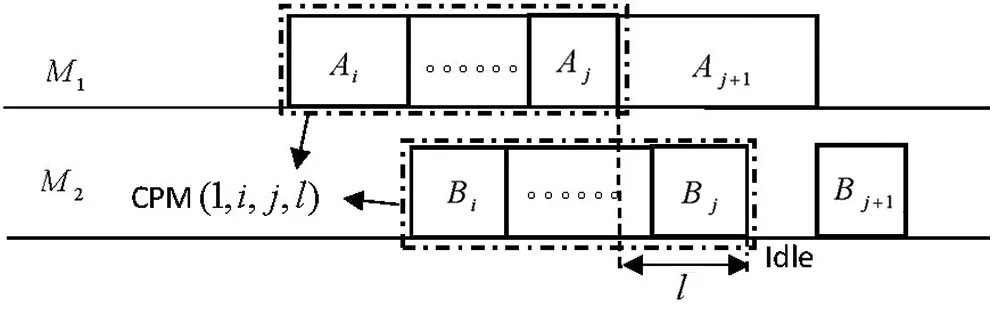Figure 2: The continuous processing module(1,i,j,l)

It is easy to see that CPM(m,i,j,l) can be constructed by CPM(m,i,j1,l) using two different methods (as shown in Fig. 3). The first method to get CPM(m,i,j,l) is to add job JjV1 to CPM(m,i,j1,l) (see Fig. 3a). And the second method to get CPM(m,i,j,l) is to add job JjV2 to CPM(m,i,j1,l) (see Fig. 3b). We assume that f(m,i,j,l) is the minimum load generated by the continuous processing module on machine M1 composed of job subset {Ji,Ji+1,,Jj}.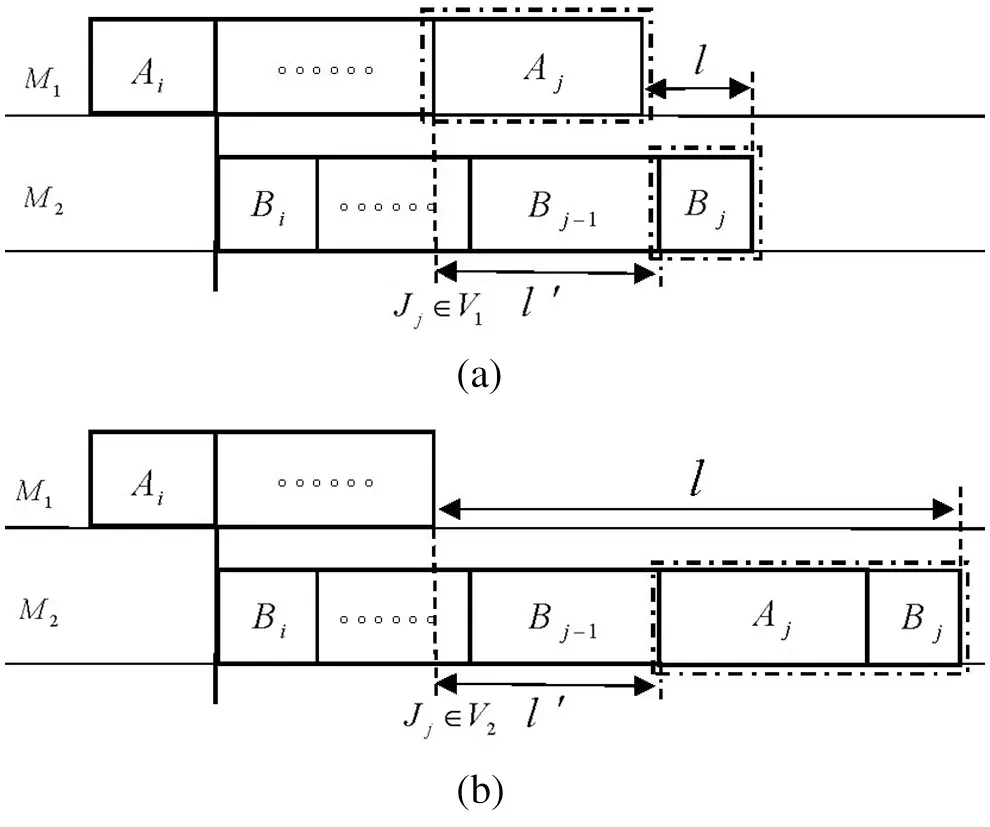Figure 3: The two ways from CPM(1,i,j1,l) to CPM(1,i,j,l) as shown in (a) and (b)

For the convenience of narration, a symbolic function is defined below:

Definition 3.2 Symbolic function σ(m) is defined as follows:

σ(m)={0,whenm=1;1,whenm=2;m{1,2}.

When i<j, according to the definition of CPM(m,i,j,l), it is easy to get the following formulas hold:

aiσ(m)+t=ijbtt=i+1jatl;(1)

laiσ(m)+t=ijbt+t=i+1jat;(2)

lbj.(3)

In the following discussion, we let

l_=max{bj,aiσ(m)+t=ijbtt=i+1jat},

l¯=aiσ(m)+t=ijbt+t=i+1jat.

Obviously, in the sub-schedule composed of job subset {Ji,Ji+1,,Jj}, the difference in completion time between two machines l must be obtained in the interval [l_l¯]. The maximum completion time of CPM(m,i,j,l) is f(m,i,j,l)+l. So, when l is fixed, minimizing the maximum completion time is equal to minimizing f(m,i,j,l). Therefore the optimal continuous processing module is obtained when f(m,i,j,l) is minimum. The dynamic programming algorithm for calculating the load f(m,i,j,l) of the optimal CPM(m,i,j,l) on machine M1 is given below:

Dynamic Programming Algorithm CPM(C):

Initial Conditions:

f(m,i,j,l)={ai,whenm=1,i=j,l=bi;0,whenm=2,i=j,l=ai+bi;+otherwise.(4)

Recurrence Relation:

The parameters m,i,j,l respectively satisfy m{1,2}, 1i<jn, l_ll¯.

(1)   When Aj is processed on M1 (see Fig. 3a),

f1=f(m,i,j1,l+ajbj)+aj;

(2)   When Aj is processed on M2 (see Fig. 3b),

f2={f(m,i,j1,lajbj)whenlaj+bj;+otherwise.

Recurrence Formula: f(m,i,j,l)=min{f1,f2}.

The initial conditions and recurrence formula in dynamic programming algorithm CPM(C) are clearly valid. So we mainly analyze the recurrence relation below. Given a set of required parameters m,i,j,l, the load generated by the optimal CPM(m,i,j,l) on M1f(m,i,j,l) can be obtained by two different methods. The first method is to put task Aj on M1 and task Bj on M2 (as shown in Fig. 3a). So we have l+bj=l+aj which implies l=l+ajbj, subject to laj, i.e., lbj. According to the value range of l, we have lbj always holds. Therefore the relation (1) in Recurrence Relation holds. The other method is to put Aj and Bj both on M2 (as shown in Fig. 3b). So we have l=l+aj+bj which implies l=lajbj, subject to l0, i.e., laj+bj. Therefore the relation (2) in Recurrence Relation holds.

After the optimal continuous processing modules are constructed, the whole schedule can be constructed by recursively connecting these optimal continuous processing modules. It should be noted that every two continuous optimal continuous processing modules are separated by an idle time on M2. First, we let the Partial Schedule (m,i) be a sub-schedule of the job set {Ji,Ji+1,,Jn}, denoted by PS(m,i), where the first job JiVm,m{1,2}. Then let g(m,i) be the minimum makespan of PS(m,i). Next, we give a dynamic programming algorithm to calculate the makespan g(m,i) of the optimal PS(m,i). For the convenience of narration, we set up a virtual job Jn+1 with processing time an+1=bn+1=+.

Dynamic Programming Algorithm DP(C):

Initial Conditions:

g(m,n+1)=0,m=1,2;

Recurrence Relation:

The parameters m,i, respectively, satisfy m{1,2}, 1in.

g={f(m,i,j,l)+ljn+g(1,j+1)whenl<aj+1;+otherwise.(5)

Recurrence Formula: g(m,i)= min ijn l _ l l ¯   {g} .

Target Value: minCmax=minm{1,2}{g(m,1)}.

The initial conditions, recurrence formula, and target value in dynamic programming algorithm DP(C) are clearly valid. So we also mainly analyze the recurrence relation below. When the optimal CPM(m,i,j,l) is given, PS(m,i) can be structured by the optimal PS(m,j+1). Since there is an idle time between CPM(m,i,j,l) and PS(m,j+1), according to Proposition 2.1, we can get m is equal to 1 in PS(m,j+1), i.e., task Aj+1 is processed on M1 as shown in Fig. 4. It is easy to get g(m,i)=minf(m,i,j,l)+g(1,j+1) when i<n, and g(m,i)=minf(m,i,j,l)+g(1,j+1)+l when i=n. According that there is an idle time between job Jj and Jj+1, l<aj+1 must be satisfied. So Eq. (5) holds.Figure 4: From CPM(m,i,j,l) and PS(m,j+1) to PS (m,i)

Theorem 3.3 Dynamic programming algorithm DP(C) is a pseudo-polynomial time algorithm, and its time complexity is O(n2i=1nai).

Proof: Firstly, we consider the time complexity of solving the optimal continuous processing module. It takes O(i=1nai) time to search l in the interval [l_l¯] and separately takes n time to search i,j in the interval [1n]. But m only has two cases. So solving the optimal continuous processing module need O(n2i=1nai) time by algorithm CB(C). In algorithm DP(C), the recursive formula needs O(ni=1nai) times of cyclic search, and each calculation of Eq. (5) requires O(n) steps. Since algorithm DP(C) only uses the calculation results of algorithm CB(C) every time, but there is no nested loop, the time complexity of algorithm DP(C) is O(n2i=1nai). So Theorem 3.3 holds.

Since SHFSFC has a pseudo-polynomial time algorithm, SHFSFC is ordinary NP-hard. That is, the following theorem holds.

Theorem 3.4 Problem SHFSFC can be solved in O(n2i=1nai) time, which implies it is NP-hard in the ordinary sense.

4  A Dynamic Programming Algorithm of Problem SHFSFW

This section will construct a dynamic programming algorithm for problem SHFSFW using a method similar to Section 3. First, we construct the optimal continuous processing modules and calculate the objective value of every optimal continuous processing module by a dynamic programming algorithm. Then, we use optimal continuous processing modules to construct an optimal partial schedule. Finally, the objective of the optimal schedule is calculated by backward recursion. However, the goal of SHFSFW is to minimize the total weighted completion time, which is different from makespan. It has no direct relationship with the load of the jobs on machine M1. So, we need to add a parameter h that represents the load of the jobs on M1 for constructing the continuous processing module. So we use five elements m,i,j,h,l instead of four elements in Section 3 to structure the continuous processing module. The strict definition of the continuous processing module for SHFSFW is given below.

Definition 4.1 A sub-schedule consisting of a job subset {Ji,Ji+1,,Jj} is called a continuous processing module of Problem SHFSFW if it satisfies the following conditions, denoted by five elements CPM(m,i,j,h,l).

(1)   The first task of job JiAi is processed on Mm (m=1 or 2);

(2)   No matter on M1 or M2, there is no idle time between any two continuously processed jobs;

(3)   The load generated by the subset {Ji,Ji+1,,Jj} on M1 is equal to h;

(4)   The difference between the complete time of subset {Ji,Ji+1,,Jj} on M1 and M2 is equal to l.

It is easy to see that CPM(m,i,j,h,l) can be structured by CPM(m,i,j1,h,l) using two different methods (as shown in Fig. 5). The first method to get CPM(m,i,j,h,l) is to add job JjV1to CPM(m,i,j1,h,l) (see Fig. 5a); The second method to get CPM(m,i,j,h,l) is to add job JjV2 to CPM(m,i,j1,h,l) (see Fig. 5b). We assume that f(m,i,j,h,l) is the minimum total weighted completion time of CPM(m,i,j,h,l) on machine M1 composed of job subset {Ji,Ji+1,,Jj}. Using the discussion similar to Section 3, we can get the dynamic programming algorithm about f(m,i,j,h,l) as follows.Figure 5: The two ways from CPM(1,i,j1,h,l) to CPM(1,i,j,h,l) as shown in (a) and (b)

Dynamic Programming Algorithm CPM(WC):

Initial Conditions:

f(m,i,j,h,l)={wi(ai+bi),whenm=1,i=j,h=ai,l=bi;wi(ai+bi),whenm=2,i=j,h=0,l=ai+bi;+,otherwise.

Recurrence Relation:

The parameters m,i,j,h,l respectively satisfy m{1,2}, 1i<jn, 0ht=ijat, l_ll¯.

(1)   When Aj is processed on M1 (see Fig. 5a),

f1=f(m,i,j1,haj,l+ajbj)+wj(h+l);

(2)   When Aj is processed on M2 (see Fig. 5b),

f2={f(m,i,j1,h,lajbj)+wj(h+l)whenlaj+bj;+otherwise.

Recurrence Formula: f(m,i,j,h,l)=min{f1,f2}.

Next, we define the Partial Schedule (m,i) of SHFSFW. Let Partial Schedule (m,i) be a sub-schedule of the job set {Ji,Ji+1,,Jn}, denoted by PS(m,i), where the first job JiVm,m{1,2}. Then let g(m,i) be the minimum total weighted completion time of PS(m,i). Next, we give a dynamic programming algorithm to calculate g(m,i). For the convenience of narration, we construct a virtual job Jn+1 with processing time an+1=+,bn+1=+.

Dynamic Programming Algorithm DP(WC):

Initial Conditions:

g(m,n+1)=0,m=1,2;

Recurrence Relation:

The parameters m,i respectively satisfy m{1,2}, 1in.

g={f(m,i,j,h,l)+g(1,j+1)+(t=j+1nwt)hwhenl<aj+1;+otherwise.(6)

Recurrence Formula: g(m,i)= min ijn l _ l l ¯ 0h t=i j a t {g} .

Target Value: minwiCi=minm{1,2}{g(m,1)}.

The initial conditions, recurrence formula, and target value in dynamic programming algorithm DP(WC) are clearly valid. So we also mainly analyze the recurrence relation below. Once the optimal CPM(m,i,j,h,l) is given, PS(m,i) can be obtained through the optimal PS(m,j+1). For there is an idle time between CPM(m,i,j,h,l) and PS(m,j+1), according to Proposition 2.1, we have m is equal to 1 in PS(m,j+1). We can easily have g(m,i)=f(m,i,j,h,l)+g(1,j+1)+(t=j+1nwt)h. According that there is an idle time between Jj and Jj+1, l<aj+1 must be satisfied. So Eq. (6) holds.

Through the analysis similar to Theorem 3.3 in Section 3, we can obtain the following theorem on the time complexity of dynamic programming algorithm DP(WC).

Theorem 4.2 Dynamic programming algorithm DP(WC) is a pseudo-polynomial time algorithm, and its time complexity is O(n2(i=1nai)2).

Similar to Theorem 3.4, we can have the following theorem.

Theorem 4.3 Problem SHFSFC can be solved in O(n2(i=1nai)2) time, which implies it is NP-hard in the ordinary sense.

5  Analysis of Algorithm Effect Based on Numerical Experiments

5.1 Time Complexity Analysis

We analyze the computational complexity of two problems, design dynamic programming algorithms for them, and calculate the time complexity of the algorithms in Sections 24. These results are summarized in Table 1.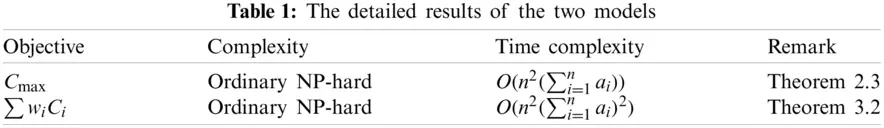We took SHFSFC as an example to test the operation efficiency of the dynamic programming algorithms designed in this paper through numerical experiments. The numerical experiments were carried out in Matlab R2017 on a laptop with built-in an Intel Core i5 8250u CPU, 8 GB LPDDR3 RAM, and Windows 10. The processing time of each flexible task ai was obtained by a random partition of a given number i=1nai into n values. The processing time of bi was produced as a uniformly distributed random number within [0,10]. The experiments were performed for 10n230 with an interval of 20 and 100i=1nai1000 with an interval of 100. We conducted a total of 12×10=120 experiments with different combinations of n and i=1nai. And we generated 30 random test instances for each experiment. The average running results of all experiments are listed in Table 2, where the top row represents i=1nai, the leftmost column represents n, and the unit of the data in the table is in seconds.

It can be seen from Table 2 that even if n reaches 230 and the total processing time of all flexible tasks reaches 1000, the time used by algorithm DP(C) only needs less than 85 s. Therefore, we can conclude that the actual effect of the algorithm is entirely acceptable.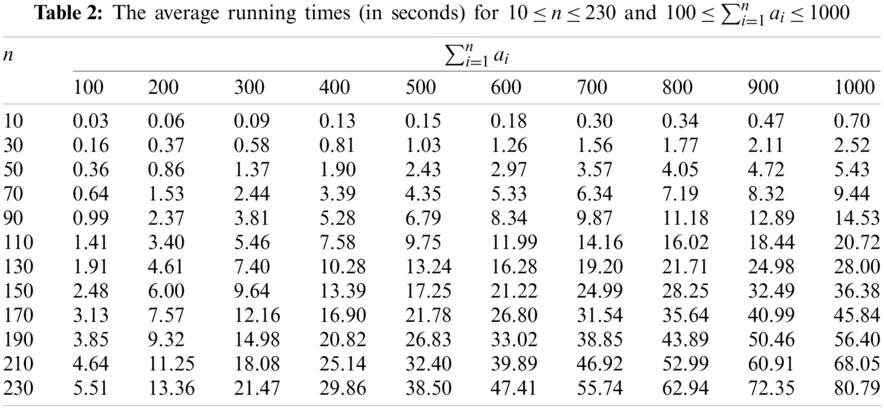Using the data in Table 2, we can discuss the relationship between the running time of the algorithm and the total processing time of all flexible tasks when there are fewer jobs (n=10), a lot of jobs (n=110), and a large number of jobs (n=210) (as shown in Fig. 6). It can be got from Fig. 6 that: (1) The running time of algorithm DP(C) increases with the increase of the total processing time of all flexible tasks i=1nai; (2) The more the number of jobs, the faster the running time of the algorithm increases with the increase of i=1nai; (3) When n is given, the growth rate of the algorithm processing time is similar, i.e., the running time of the algorithm is nearly linear with i=1nai.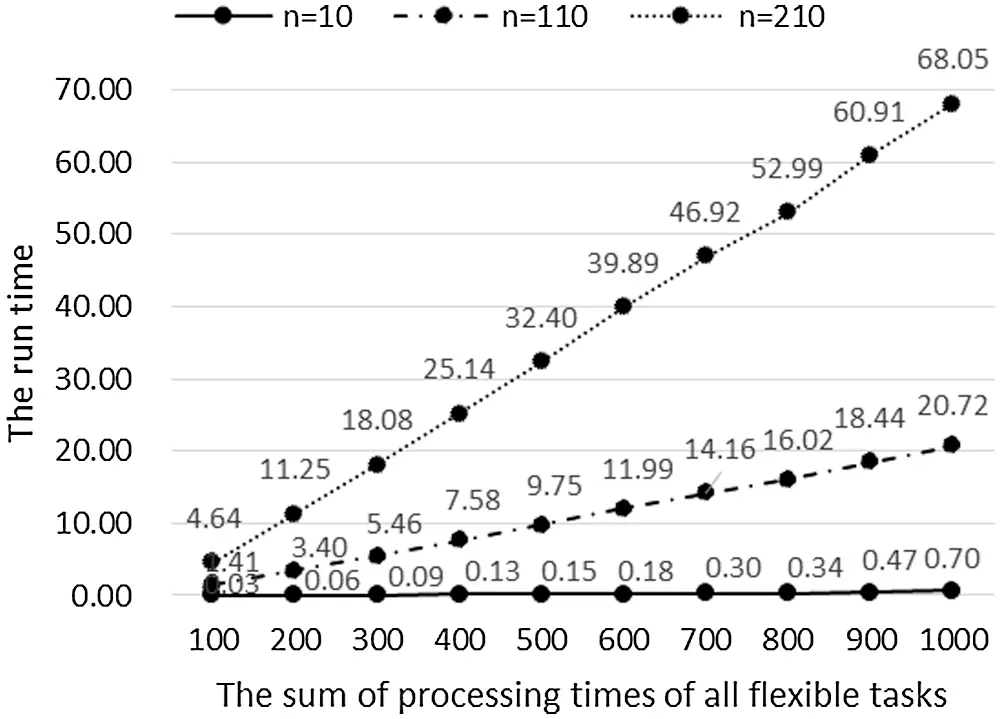Figure 6: When n=10, 110, 210, the running time changes with i=1nai

Similarly, the data in Table 2 is used to analyze the relationship between the running time of the algorithm and the number of jobs when the total processing time of all flexible tasks is small (i=1nai=100), large (i=1nai=500), and huge (i=1nai=1000) (as shown in Fig. 7). It can be got from Fig. 7 that: (1) The running time of the algorithm increases with the increase of the number of all jobs; (2) The growth rate of the algorithm running time becomes faster with the increase of the number of all jobs; (3) The larger the total processing time of all flexible tasks, the faster the algorithm processing time increases with the increase of the number of all jobs.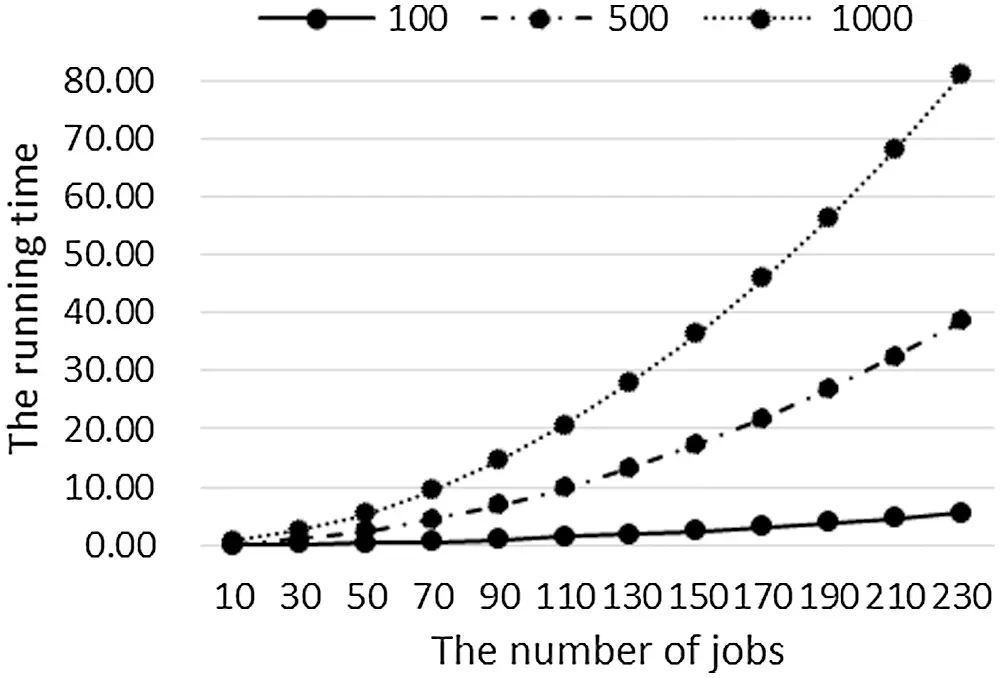Figure 7: When i=1nai=100, 500, 1000, the running time changes with n

5.2 Comparison with Other Commonly Used Algorithms

Since there is no other algorithm for the two-stage hybrid flow-shop problem studied in this paper, we compare the high-quality algorithms for a similar problem with the dynamic programming algorithms given in this paper to analyze the effect of our algorithms.

We usually design polynomial-time optimal algorithms to solve P problems . But there exist two kinds of algorithms for solving NP-hard problems. The first one is the exact algorithm, which gives the optimal solution, but the time complexity is exponential, including the enumeration algorithm, branch and bound algorithm . The second one is the heuristic algorithm, which usually gives the approximate solution of the problem, but the time complexity is polynomial, including the genetic algorithm [20,32], (Iterated) green algorithm , fireworks algorithm , artistic neural network algorithm , (discrete) harmony search algorithm , etc. These algorithms are widely used in solving various scientific and engineering problems. From the analysis of the existing literature, it is found that the branch and bound algorithm is the most commonly used and relatively practical algorithm in the accurate solution of hybrid flow-shop problems [31,37]. Regarding the heuristic algorithm, the research in literature  shows that a discrete harmony search algorithm has advantages compared with a genetic algorithm, greedy algorithm, and harmony search algorithm, both in running time and the average relative percentage deviation, in solving hybrid flow-shop problems. The average Relative Percentage Deviation (denoted by RPD) is usually used to measure the approximation of heuristic algorithms , and its specific definition is as follows: RPD=CACC×100%, in which CA is the objective value calculated by Algorithm A and C is the optimal objective value which can be got by the exact algorithm.

We still took problem SHFSFC as an example to compare dynamic programming algorithm DP(C) (the algorithm given by us), the branch and bound algorithm (given by Moursli et al. , denoted by B&B), and the discrete harmony search algorithm (given by Zini et al. , denoted by DHS) regarding the running time and the average relative percentage deviation.

The numerical experiments were carried out in the same software and hardware environment as in Section 5.1. The time complexity of B&B and DHS is independent of i=1nai but only related to the number of jobs. So, we only conducted grouping experiments according to n to unify the comparison standards. We considered the efficiency (running time) and performance (RPD) of the three algorithms in the small-scale-jobs case (5n50) and the large-scale-jobs case (100n300). The numerical experiments were carried out for 5n50 with an interval of 5 in the small-scale-jobs case and 100n300 with an interval of 50 in the large-scale-jobs case. The processing time of each task ai (or bi) was produced as a uniformly distributed random number within [0,10]. Twenty random instances for every experiment were produced. The average values of running time and RPDs were selected as the experimental results. The average running time of each algorithm under the two experimental conditions is shown in Table 3.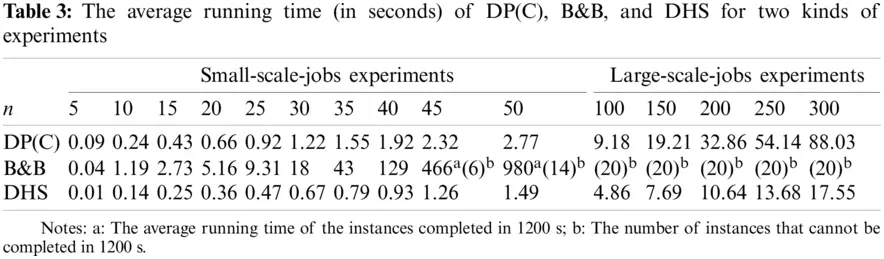As shown in Table 3, DP(C) and DHS can complete the operation in 100 s in both two cases. In particular, DHS can be completed in 20 s, showing its advantage in time complexity. However, for B&B, when the number of jobs reaches 45, some instances cannot be completed in 1200 s; when the number of jobs reaches 50%, 70% of the instances cannot be completed in 1200 s; and all large-scale-jobs experiments cannot be completed in 1200 s.

The relationship between running time and n of each algorithm in the small-scale-jobs case is shown in Fig. 8. For this case, we can get that: (1) DHS has the most advantage in time complexity, followed by DP(C), while B&B has obvious disadvantages; (2) The running time of each algorithm increases with the increase of the number of jobs and one of B&B increases much faster than the other two algorithms.Figure 8: The average running time of DP(C), B&B, and DHS for small-scale-jobs experiments

Similarly, using the data in Table 3, the relationship between running time and n of each algorithm in the large-scale-jobs case is shown in Fig. 9. Since B&B cannot complete the operation within 1,200 s, only DHS and DP(C) are compared here. For this case, we can get that: (1) DHS has obvious advantages in time complexity, and the running time of each algorithm is within the acceptable range; (2) With the increase of n, the running time of each algorithm increases. The growth rate of the running time of DP(C) increases with the increase of n, while the growth rate of DHS is relatively flat.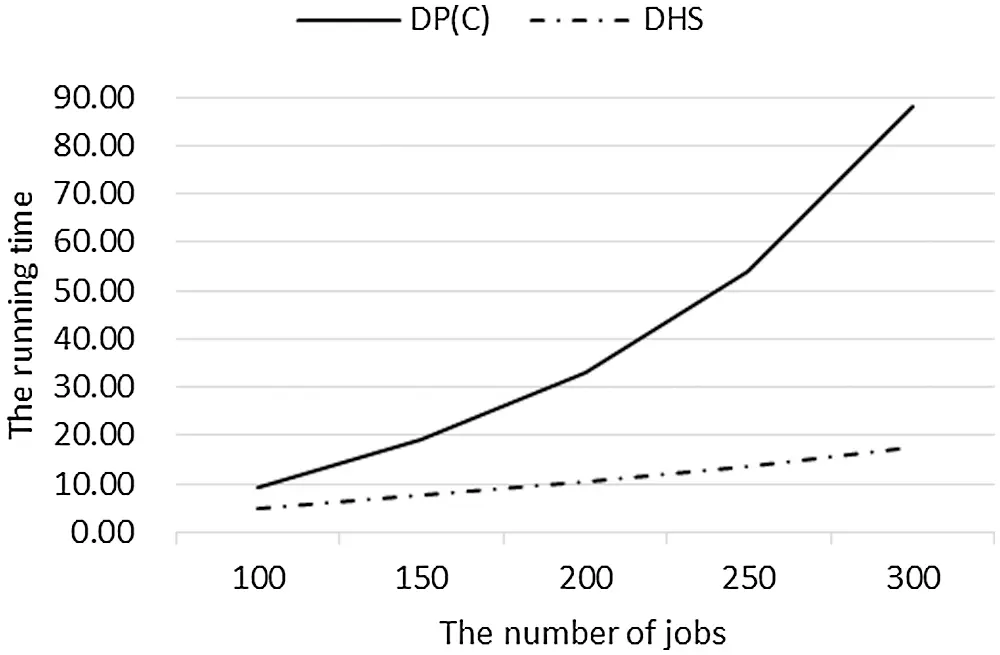Figure 9: The average running time of DP(C) and DHS for large-scale-jobs experiments

Table 4 shows the results of the three algorithms on the relative percentage deviation under small-scale-jobs experiments and large-scale-jobs experiments.

Using the data in Table 4, we plot the relationship between the average RPD of algorithm DHS and the number of jobs in Fig. 10.

From Fig. 10, we can get that: (1) Since DP(C) and B&B are both exact algorithms, their average RPDs are 0. The average RPD of DHS is large in the small-scale-jobs case and decreases rapidly with the increase of the number of jobs, which means that the accuracy of DHS increases quickly with the rise of the number of jobs. However, in the small-scale-jobs case, the difference between the solution of DHS and the optimal solution is more than 25%, especially when the number of jobs is less than 15, the error is more than 50%; (2) In the large-scale-jobs case, with the increase of n, the reduction speed of the average RPD slows down. In all large-scale-jobs experiments, the average RPD is above 0.1. Even if n reaches 300, the gap between it and the optimal solution is still 12%.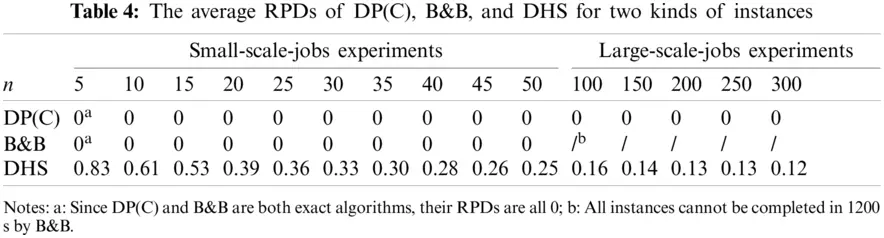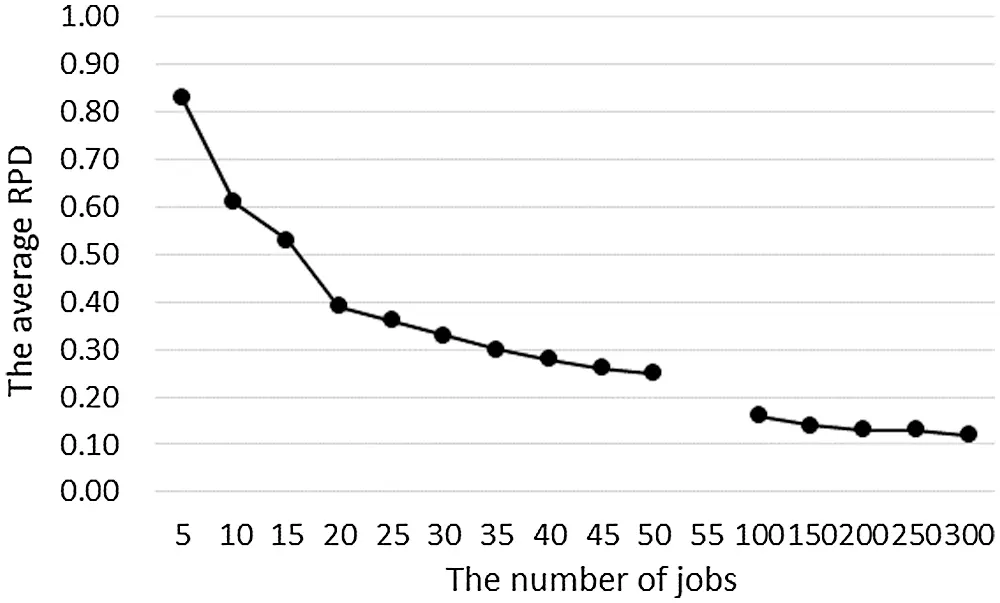Figure 10: The average RPD of DHS changes with the number of jobs for two kinds of experiments

5.3 Summary of Comparison

According to the comparative analysis of numerical experimental results in Sections 5.1 and 5.2, we can get the following results:

(1)   From the perspective of algorithm time complexity, DHS has advantages, followed by DP(C). The gap between the two can be ignored in the small-scale-jobs case. Although there is a certain gap in the large-scale-jobs case, DP(C) is still within the acceptable range. B&B has significant disadvantages in running time, and when the number of jobs is greater than 45, its running time becomes unacceptable;

(2)   From the perspective of algorithm accuracy, DP(C) and B&B are both exact algorithms so that they can give the optimal solution. But B&B cannot provide the optimal solution within the time limit when the number of jobs is immense. DHS is a heuristic algorithm. In the small-scale-jobs case, the accuracy is low, and the gap with the optimal solution is more than 25%. In the large-scale-jobs case, the accuracy is improved, but the gap with the optimal solution is still more than 12%;

(3)   The running time of DP(C) is small, and the accuracy is better than DHS 50% in the small-scale-jobs case. Although there is a certain gap between DP(C) and DHS, the running time of DP(C) is still in the acceptable range. And the algorithm accuracy is better than DHS 12% in the large-scale-jobs case.

6  Conclusions

This paper studies two two-stage hybrid flow-shop problems with two machines and fixed processing sequences, widely used in shared manufacturing, stock control systems, and other manufacturing areas. Two objectives of minimizing makespan and total weighted completion time are considered. For these two models, we first show they are both ordinary NP-hard, present a dynamic programming algorithm for each model, and analyze the time complexity of each algorithm. Then, the relationship between the running time and the combination of the total processing time of the flexible tasks and the number of jobs is obtained by numerical experiments. Finally, the advantages and disadvantages of the dynamic programming algorithms presented in this paper are compared with the exact algorithm B&B and the heuristic algorithm DHS, which have advantages in solving hybrid flow-shop problems.

In future research, we will study the polynomial-time approximation algorithm with a smaller worst-case ratio for this problem and extend the dynamic programming algorithm's design method given in this paper to other scheduling problems with fixed processing sequences.

Acknowledgement: This work was partially supported by the Zhejiang Provincial Philosophy and Social Science Program of China (Grant No. 19NDJC093YB), the National Social Science Foundation of China (Grant No. 19BGL001), the Natural Science Foundation of Zhejiang Province of China (Grant No. LY19A010002) and the Natural Science Foundation of Ningbo of China (The design of algorithms and cost-sharing rules for scheduling problems in shared manufacturing, Acceptance No. 20211JCGY010241).

Funding Statement: This study was funded by the Zhejiang Provincial Philosophy and Social Science Program of China (Grant No. 19NDJC093YB), Recipient: Qi Wei. And the National Social Science Foundation of China (Grant No. 19BGL001), Recipient: Qi Wei. And the Natural Science Foundation of Ningbo of China (Acceptance No. 20211JCGY010241), Recipient: Qi Wei. And the Natural Science Foundation of Zhejiang Province of China (Grant No. LY19A010002), Recipient: Yong Wu.

Conflicts of Interest: The authors declare that they have no conflicts of interest to report regarding the present study.

## References

1. Vairaktarakis, G., & Lee, C. Y. (2004). Analysis of algorithms for two-stage flowshops with multi-processor task flexibility. Naval Research Logistics, 51, 44-59. [Google Scholar] [CrossRef]
2. Wei, Q., & He, Y. (2005). A two-stage semi-hybrid flowshop problem in graphics processing. Applied Mathematics: A Journal of Chinese Universities B, 20, 393-400. [Google Scholar] [CrossRef]
3. Zhong, W. Y., & Shi, Y. (2018). Two-stage no-wait hybrid flowshop scheduling with inter-stage flexibility. Journal of Combinatorial Optimization, 35(1), 108-125. [Google Scholar] [CrossRef]
4. Li, Y., Li, X., & Gao, L. (2020). Review on hybrid flow shop scheduling problems. China Mechanical Engineering, 31(23), 2798. [Google Scholar] [CrossRef]
5. Lin, B. M. T., & Hwang, F. J. (2011). Total completion time minimization in a 2-stage differentiation flowshop with fixed sequences per job type. Information Processing Letters, 111(5), 208-212. [Google Scholar] [CrossRef]
6. Yu, C., Xu, X., Yu, S., Sang, Z., & Yang, C. (2020). Shared manufacturing in the sharing economy: Concept, definition and service operations. Computers & Industrial Engineering, 146, 106602. [Google Scholar] [CrossRef]
7. Jiang, Y. W., & Wei, Q. (2011). An improved algorithm for a hybrid flow-shop problem in graphics processing. Acta Automatica Sinica, 37(11), 1381-1386. [Google Scholar] [CrossRef]
8. Wei, Q., & Wu, Y. (2020). Dynamic programming algorithms for two-machine hybrid flow-shop scheduling with a given job sequence and deadline. IEEE Access, 8, 89964-89975. [Google Scholar] [CrossRef]
9. Zhang, M., Lan, Y., & Han, X. (2020). Approximation algorithms for two-stage flexible flow shop scheduling. Journal of Combinatorial Optimization, 39(1), 1-14. [Google Scholar] [CrossRef]
10. Peng, A. Z., Liu, L. C., & Lin, W. F. (2021). Improved approximation algorithms for two-stage flexible flow shop scheduling. Journal of Combinatorial Optimization, 41(1), 28-42. [Google Scholar] [CrossRef]
11. Wang, S., Kurz, M., Mason, S. J., & Rashidi, E. (2019). Two-stage hybrid flow shop batching and lot streaming with variable sublots and sequence-dependent setups. International Journal of Production Research, 57(22), 6893-6907. [Google Scholar] [CrossRef]
12. Tan, Y., Monch, L., & Fowler, J. W. (2017). A hybrid scheduling approach for a two-stage flexible flow shop with batch processing machines. Journal of Scheduling, 21(2), 209-226. [Google Scholar] [CrossRef]
13. Dong, J., Pan, H., Ye, C., Tong, W., & Hu, J. (2020). No-wait two-stage flowshop problem with multi-task flexibility of the first machine. Information Sciences, 544, 25-38. [Google Scholar] [CrossRef]
14. Wang, S., Wang, X., & Yu, L. (2020). Two-stage no-wait hybrid flow-shop scheduling with sequence-dependent setup times. International Journal of Systems Science: Operations & Logistics, 7(3), 291-307. [Google Scholar] [CrossRef]
15. Shao, W., Shao, Z., & Pi, D. (2021). Effective constructive heuristics for distributed no-wait flexible flow shop scheduling problem. Computers & Operations Research, 136, 105482. [Google Scholar] [CrossRef]
16. Feng, X., Zheng, F., & Xu, Y. (2016). Robust scheduling of a two-stage hybrid flow shop with uncertain interval processing times. International Journal of Production Research, 54(12), 1-12. [Google Scholar] [CrossRef]
17. Ahonen, H., & Alvarenga, A. G. (2017). Scheduling flexible flow shop with recirculation and machine sequence-dependent processing times: Formulation and solution procedures. The International Journal of Advanced Manufacturing Technology, 89(1–4), 765-777. [Google Scholar] [CrossRef]
18. Lei, D., & Wang, T. (2020). Solving distributed two-stage hybrid flowshop scheduling using a shuffled frog-leaping algorithm with memeplex grouping. Engineering Optimization, 52(9), 1461-1474. [Google Scholar] [CrossRef]
19. Cai, J., Zhou, R., & Lei, D. (2020). Fuzzy distributed two-stage hybrid flow shop scheduling problem with setup time: Collaborative variable search. Journal of Intelligent & Fuzzy Systems, 38(3), 3189-3199. [Google Scholar] [CrossRef]
20. Rolf, B., Reggelin, T., Nahhas, A., Müller, M., Lang, S. (2020). Scheduling jobs in a two-stage hybrid flow shop with a simulation-based genetic algorithm and standard dispatching rules. Winter Simulation Conference, pp. 1584–1595. Florida, USA.
21. Wang, S., Wang, X., Chu, F., & Yu, J. B. (2020). An energy-efficient two-stage hybrid flow shop scheduling problem in a glass production. International Journal of Production Research, 58(8), 2283-2314. [Google Scholar] [CrossRef]
22. Shafransky, Y. M., & Strusevich, V. A. (1998). The open shop scheduling problem with a given sequence of jobs on one machine. Naval Research Logistics, 45(7), 705-731. [Google Scholar] [CrossRef]
23. Hwang, F. J., Kovalyov, M. Y., & Lin, B. M. T. (2012). Total completion time minimization in two-machine flow shop scheduling problems with a fixed job sequence. Discrete Optimization, 9(1), 29-39. [Google Scholar] [CrossRef]
24. Hwang, F. J., Kovalyov, M. Y., & Lin, B. M. T. (2014). Scheduling for fabrication and assembly in a two-machine flowshop with a fixed job sequence. Annals of Operations Research, 217(1), 263-279. [Google Scholar] [CrossRef]
25. Lin, B. M. T., Hwang, F. J., & Kononov, A. V. (2016). Relocation scheduling subject to fixed processing sequences. Journal of Scheduling, 19(2), 153-163. [Google Scholar] [CrossRef]
26. Halman, N., & Vinetz, U. (2021). An FPTAS for two performance measures for the relocation scheduling problem subject to fixed processing sequences. Optimization Letters, 2021, 1-16. [Google Scholar] [CrossRef]
27. Cheref, A., Agnetis, A., Artigues, C., & Billaut, J. C. (2017). Complexity results for an integrated single machine scheduling and outbound delivery problem with fixed sequence. Journal of Scheduling, 20(6), 681-693. [Google Scholar] [CrossRef]
28. Cheng, T. C. E., Kravchenko, S. A., & Lin, B. M. T. (2019). Server scheduling on parallel dedicated machines with fixed job sequences. Naval Research Logistics, 66(4), 321-332. [Google Scholar] [CrossRef]
29. Cheng, T. C. E., Kravchenko, S. A., & Lin, B. M. T. (2020). Complexity of server scheduling on parallel dedicated machines subject to fixed job sequences. Journal of the Operational Research Society, 2020, 1-4. [Google Scholar] [CrossRef]
30. Ji, M., Zhang, W. Y., Liao, L. J., Cheng, T. C. E., & Tan, Y. Y. (2019). Multitasking parallel-machine scheduling with machine-dependent slack due-window assignment. International Journal of Production Research, 57(6), 1667-1684. [Google Scholar] [CrossRef]
31. Moursli, O., & Pochet, Y. (2000). A branch-and-bound algorithm for the hybrid flowshop. International Journal of Production Economics, 64, 113-125. [Google Scholar] [CrossRef]
32. Nirmal Kumar, S. J., Ravimaran, S., & Alam, M. M. (2020). An effective non-commutative encryption approach with optimized genetic algorithm for ensuring data protection in cloud computing. Computer Modeling in Engineering & Sciences, 125(2), 671-697. [Google Scholar] [CrossRef]
33. Shao, W., Shao, Z., & Pi, D. (2020). Modeling and multi-neighborhood iterated greedy algorithm for distributed hybrid flow shop scheduling problem. Knowledge-Based Systems, 194, 105527. [Google Scholar] [CrossRef]
34. Pang, X., Xue, H., Tseng, M. L., Lim, M. K., & Liu, K. (2020). Hybrid flow shop scheduling problems using improved fireworks algorithm for permutation. Applied Sciences, 10(3), 1174. [Google Scholar] [CrossRef]
35. Karaci, A., Yaprak, H., Ozkaraca, O., Demir, I., & Simsek, O. (2019). Estimating the properties of ground-waste-brick mortars using DNN and ANN. Computer Modeling in Engineering & Sciences, 118(1), 207-228. [Google Scholar] [CrossRef]
36. Zini, H., Elbernoussi, S. (2017). Minimizing makespan in hybrid flow shop scheduling with multiprocessor task problems using a discrete harmony search. IEEE International Conference on Computational Intelligence & Virtual Environments for Measurement Systems & Applications, pp. 177–180. Annecy, France.
37. Wang, S., Liu, M., & Chu, C. (2015). A branch-and-bound algorithm for two-stage no-wait hybrid flow-shop scheduling. International Journal of Production Research, 53(4), 1143-1167. [Google Scholar] [CrossRef]
38. Priya, A., & Sahana, S. K. (2020). Multiprocessor scheduling based on evolutionary technique for solving permutation flow shop problem. IEEE Access, 8, 53151-53161. [Google Scholar] [CrossRef]This work is licensed under a Creative Commons Attribution 4.0 International License, which permits unrestricted use, distribution, and reproduction in any medium, provided the original work is properly cited.